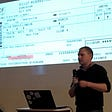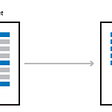# Palindrome Program Explained

C-Programs to find a palindrome

A Palindrome is a word, sequence or phrase that reads the same forward as it does backwards, for example words like ‘dad’, ‘mom’, ‘madam’ or numbers like ‘11’, ‘121’, or ‘99099’. In this article we will create two palindrome programs. One palindrome program will check if a word is a palindrome or not, and the second program will check if a number is a palindrome or not. The idea is to not only properly identify a palindrome, but to also do it in something called Big O of n time O(n). If you are not familiar with Big O notation, that just basically mean we will only use one loop and no inner for looping through the code, although technically we could use many loops and the program could still be O(n) as long as none of the loops are within each other.

# Palindrome Program I (Words):

Description: This program will determine if a word or a string is a palindrome. You can find the code on my GitHub.

Example #1:
Input: “MOM”
Output: Yes, MOM is a palindrome because the word reversed is the same.

Example #2:
Input : “TOM”
Output: No, Tom is NOT a palindrome

# How The Algorithm Works

How to determine if a word is a palindrome or not ? Well first we will take in the word for example “MOM”. A word in programming is just a string and a string is just an array of characters so “MOM” = [‘M’,’O’,’M’].

We need to create a character array (a.k.a string) variable to store our string/ word in, let’s call it “word” such that word = “MOM” = [‘M’,’O’,’M’]. Note the variable word has length = 3, because it holds 3 characters, this is important as we will use this later. Let’s create a variable called “len” and set it equal to the string word length to get len= strlen(word) = 3;

If a word is a palindrome then the starting letter and the ending letter should be the same, in this case for “MOM”, the starting letter is at position ‘0’ of the array such that word = ‘M’ , and the last character is at position ‘2’ such that word = ‘M’, if we are clever and we are, then we can rewrite this as word[len-1] = word[3 -1] = word.

We can now check the very last letter and the first letter in our array, if they match then we check the next letter.

For example if we had a string such as str = “abcda”, then we would do the following:

Does str at position 0 equal str at position 4 ? If yes then continue and check the next characters. str = ‘a’ and str = ‘a’ so yes.

Does str at position 1equal str at position 3? If yes then continue and check the next characters. str = ‘b’ and str = ‘d’ so no. Therefore the word isn’t a palindrome.

if str != str[strlen-1]
then the string is not a palindrome

if
the above statement is not true (e.g. the characters that we are comparing are the same)
then increase our left most index ‘0’ , and decrease our Right most index ‘strlen-1’

Continue this until the left most index >= Right most index

`//Description: This program determines if a word or string is a palindrome/*Example1:INPUT: "MOM"OUTPUT: Yes, MOM is a palindrome because the word reversed is the sameExample2:INPUT: "TOM" OUTPUT: No, TOM is NOT a palindrome*/#include<stdio.h>#include<string.h> // strlen()void isPalindrome(char str[]);int main(){		isPalindrome("MOM");	isPalindrome("M");	return 0;}/*    EX: "MOM" = str['M','O','M'], strlen = 3    if str != str[strlen-1] then the string is not a palindrome     if the above statement is not true (e.g. the characters that we are comparing are the same)    then increase our left most index '0' , and decrease our Right most index 'strlen-1'        Continue this until the left most index >= Right most index*/void isPalindrome(char str[]){		int lm = 0;//left most index	int rm = strlen(str) - 1;//right most index		while(rm > lm){				if(str[lm++] != str[rm--]){						printf("No, %s is NOT a palindrome \n", str);			return;		}			}		printf("Yes, %s is a palindrome because the word reversed is the same \n", str);	}`

# Palindrome Program II (Integers):

Description: This program checks if an integer is a palindrome meaning the number reversed is the same number

Example:
1) 101 reversed = 101 , 101 is a palindrome

2) 12 reversed = 21, 12 is not a palindrome

3) 11 reversed = 11, 11 is a palindrome

# How The Algorithm Works

For this algorithm we will have an original number such as ‘101’ and then we will create a reversal of that number. So we will need two variables reversedInteger and originalNumber. We then need to compare the two variables if they are equal then the number is a palindrome, and if they are not equal then the number is NOT a palindrome.

The trick is to reverse the original number. In order to reverse the original number, we will use the modular operator to get a remainder. More specifically to get the last digit from the original number, we will mod (short for modular operation)it by 10. For example if the original number was ‘101’, then 101 mod 10 = 1, because 101 / 10 = 10 with a remainder of 1.

Okay now we must go through every digit in the original number, not just the ones place, but the tens, hundreds, thousands etc, to do this we will need a loop. We can keep dividing the original number by 10 to get the next digit. For example 101/10 = 10 so now we can take the answer ‘10’ and mod it by 10 to get the middle number 10 mod 10 = 0. Next we take the new number ‘10’ and divide it by 10 to get the next number ‘1’, and we do the operation 1 mod 10 = 1.

So now to revisit everything we just talked about, first we had the original number ‘101’.

Next we took 101 and mod it by 10 to get the following:

101 mod 10 = 1

REMAINDER = 1

After that we took the original number ‘101’ and divided it by 10 to get the new number:

101 / 10 = 10

NOTE: This is integer division in the C-programming language so there will be no decimal place values. So 101/10 DOES NOT equal 10.1

Next we took the new number ‘10’ and mod it by 10 to get the following:

10 mod 10 = 0

REMAINDER = 0

After that we took the number ‘10’ and divided it by 10 to get the new number:

10 / 10 = 1

Next we took the new number ‘1’ and mod it by 10 to get the following:

1 mod 10 = 1

REMAINDER = 1

If we go back and write the remainders, we will see that if we append them we get the number ‘101’. So we need to be able to store those values in a way that they can be written in that form. We need to store the remainders in a integer variable. Well we already have two created reversedInteger and originalNumber. We will use reversedInteger.

We will set this variable reversedInteger = 0 initially.
Then when we get the remainder from the original number, we can perform the calculation below:

reversedInteger = reversedInteger * 10 + remainder;

Let’s take a look at how this will work in a loop. If our number was 101 and we got the same remainders, then we get:

1) reversedInteger = reversedInteger * 10 + 1; → 0 * 10 + 1;
2) reversedInteger = reversedInteger * 10 + 0; → 1* 10 + 0;
3) reversedInteger = reversedInteger * 10 + 1; → 10* 10 + 1;

In the first calculation reversedInteger = 1, in the second calculation reversedInteger = 10, and in the third reversedInteger = 101.

Now we need to know when to stop our loop. Well our loop only needs to run as long as the integer variable is for example ‘101’ should only run 3 times. If we divide this number by 10 three times, then we will see that the value becomes 0 (remember integer division).

So the loop will run while our original number does not equal 0 and keep dividing it by 10 each time. There is only one problem, we want to be able to compare our reversed number with our original number, so we will create another variable called n and set it equal to the original number and perform all of our calculations on it.

Now all we have to do is see if our reversed number equals our original number if it does then the number is a palindrome and if it doesn’t, then our number is not a palindrome.

You can find all of the code for this algorithm on my GitHub

Thanks for reading this article I hope its helpful to you all ! Keep up the learning, and if you would like more computer science, programming and algorithm analysis videos please visit and subscribe to my YouTube channels (randerson112358 & compsci112358 )

# Check Out the following for content / videos on Computer Science, Algorithm Analysis, Programming and Logic:

compsci112358:

Video Tutorials on Recurrence Relation:

Video Tutorial on Algorithm Analysis:
https://www.udemy.com/algorithm-analysis/

# Udemy Videos on Algortithm Analysis:

--

--

--

## More from randerson112358

Love podcasts or audiobooks? Learn on the go with our new app.

## NVIDIA Jetson Nano: Communication with industrial PLC using OPC UA## How to Hire .NET Developers## 【FFloat】Super powerful Flutter floating widget## How to Extend Geta SEO.Sitemaps in Episerver## How to Validate Your Kubernetes Cluster With Sonobuoy## How Hiscox insurance adopted agile working in a £0.5bn bet on the cloud## Apache Kafka Beginner Guide — I## Detect and Blur faces using OpenCV and Python## Minestal (Technical, Analytics, Cryptology, Layer Switch, 2FA, Data, System)## Build Own Text Summarizer with Python## My experience learning stacks in Data structure